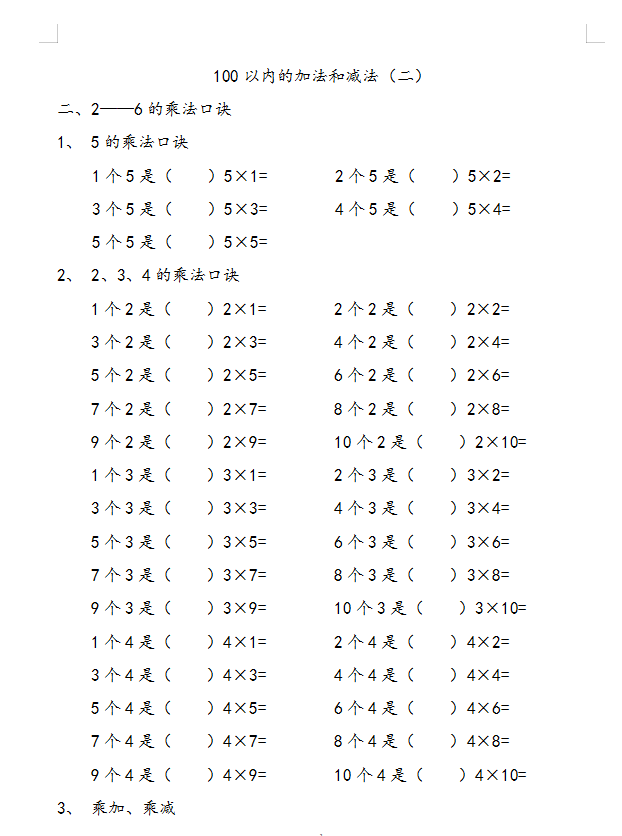100以内的加法和减法（二）

1、 5的乘法口诀

1个5是（    ）5×1=        2个5是（    ）5×2=

3个5是（    ）5×3=        4个5是（    ）5×4=

5个5是（    ）5×5=

2、 2、3、4的乘法口诀

1个2是（    ）2×1=        2个2是（    ）2×2=

3个2是（    ）2×3=        4个2是（    ）2×4=

5个2是（    ）2×5=        6个2是（    ）2×6=

7个2是（    ）2×7=        8个2是（    ）2×8=

9个2是（    ）2×9=        10个2是（    ）2×10=